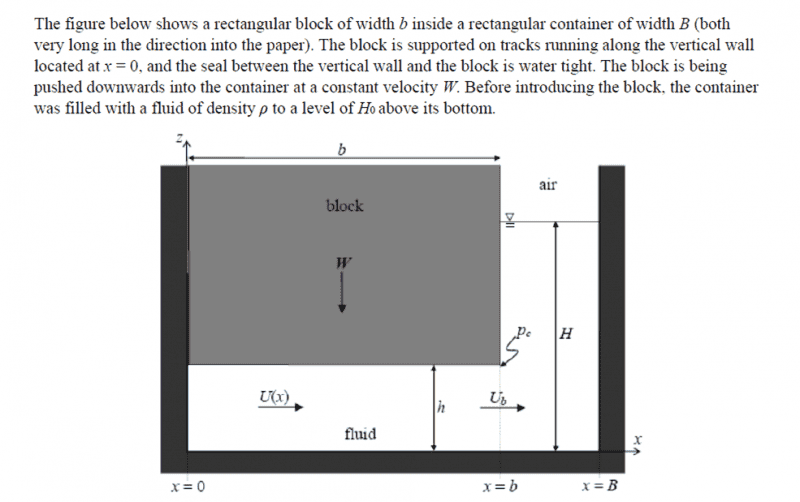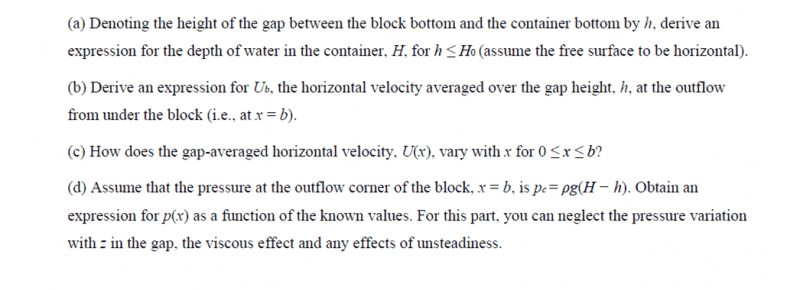# Bernoulli Equation Problem

RGG
Hey everyone ! I'm new here and found that this forum was very useful. Would really appreciate it if you could help me out with this problem ! Have been scratching my head for hours now :(

Question:Thank you very very much once again !

## Answers and Replies

proton007007
The (a) part can be solved by conserving volume of the liquid . (Think about rate of change of volume !!)

RGG
heya, A was okay actually. The main problems I have are with B onwards !

Thank you for your viewership :)

RGG
Okay, so I was told that my homework request was an unreasonable one and hence, I have looked through the forum rules. I shall abide by them ! So sorry for being ignorant.

See Above

## Homework Equations

Bernoulli Equation: V2/2g + p/dg + z = constant
V = velocity of particles flowing through that point of the streamline
g = acceleration due to gravity
p = pressure at that point of the streamline
d = density
z = elevation at that point of the streamline

## The Attempt at a Solution

(a) V = H0B = bh + (B-b)H
H=(BH0-bh)/(B-b)

(b) By conservation of volume,
Discharge, Q = W.Ab = Ub.Af
Q = W.b = Ub.h

Therefore, Ub = bW/h

(c) Since gap-averaged flow from x=0 to x=b is a well-behaved flow, dU(x)/dx = 0

(d)
At point x=b: Ub/2g + dg(H-h)/dg + h = constant

From here, I'm really not too sure how to proceed.

Any help would be greatly appreciated ! Thank you !